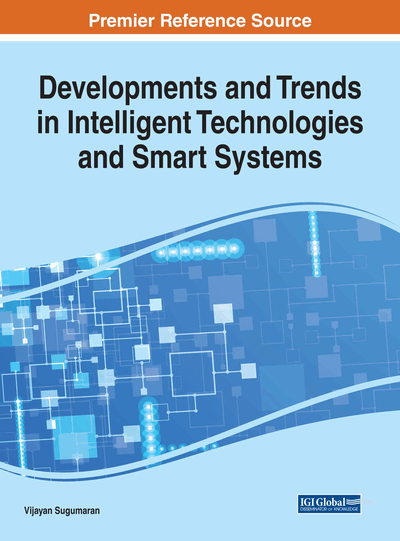# Clustering Mixed Datasets Using K-Prototype Algorithm Based on Crow-Search Optimization

Lakshmi K. (Kongu Engineering College, India), Karthikeyani Visalakshi N. (NKR Government Arts College for Women, India), Shanthi S. (Kongu Engineering College, India) and Parvathavarthini S. (Kongu Engineering College, India)
DOI: 10.4018/978-1-5225-3686-4.ch010

## Abstract

Data mining techniques are useful to discover the interesting knowledge from the large amount of data objects. Clustering is one of the data mining techniques for knowledge discovery and it is the unsupervised learning method and it analyses the data objects without knowing class labels. The k-prototype is the most widely-used partitional clustering algorithm for clustering the data objects with mixed numeric and categorical type of data. This algorithm provides the local optimum solution due to its selection of initial prototypes randomly. Recently, there are number of optimization algorithms are introduced to obtain the global optimum solution. The Crow Search algorithm is one the recently developed population based meta-heuristic optimization algorithm. This algorithm is based on the intelligent behavior of the crows. In this paper, k-prototype clustering algorithm is integrated with the Crow Search optimization algorithm to produce the global optimum solution.
Chapter Preview
Top

## Introduction

Knowledge Discovery in Databases (KDD) is an automatic, exploratory analysis and modelling of large data repositories. It is the organized as the process of identifying valid, novel, useful, and understandable patterns from large and complex data sets. Data Mining is the heart of the KDD process, involving the large number of algorithms that explore the data, develop the model and discover previously unknown patterns.

Data clustering is the process of grouping the heterogeneous data objects into homogeneous clusters such that data objects within the cluster are similar with each other and dissimilar between the other clusters.

Clustering is used in variety of fields like data mining and knowledge discovery, market research, machine learning, biology, pattern recognition, weather prediction, etc. An early specific example of the use of cluster analysis in market research is given in (Green, Frank & Robinson, 1967). A large number of cities were used as test markets and the cluster analysis was used to classify the cities into a small number of groups on the basis of variables includes city size, newspaper circulation and per capita income. It shows that cities within a group is very similar to each other, choosing one city from each group was used for selecting the test markets.

Another example is, Littmann (2000) applies cluster analysis to the daily occurrences of several surface pressures for weather in the Mediterranean basin, and finds the groups that explain rainfall variance in the core Mediterranean regions. Liu and George (2005) use fuzzy k-means clustering to account for the spatiotemporal nature of weather data in the South-Central USA. Kerr and Churchill (2001) investigate the problem of clustering tools applied to gene expression data.

There are number of clustering algorithms are available for grouping the instances of the same type. The clustering algorithms are categorized into Partitional clustering algorithms, Hierarchical clustering algorithms, Density-Based clustering algorithms and Grid-Based clustering algorithms. Partitional clustering algorithms form the clusters by partition the data objects into groups. Hierarchical clustering algorithms form the clusters by the hierarchical decomposition of data objects.

The partitional clustering algorithms include k-means, k-modes, k-medoids and k-medians. The hierarchical clustering algorithms can be classified as single linkage and complete linkage, agglomerative algorithms. Density based clustering algorithms can be listed as DBSCAN, DENCLUE, OPTICS. The grid based clustering algorithms include GRIDCLUS, BANG and STING.

The k-means algorithm handles the large amount of data objects but it handles numeric type data objects. Huang introduced the two extensions of the k-means clustering algorithm. First extension is the k-modes clustering algorithm (Huang, 1997a) and second extension is the k-prototype clustering algorithm (Huang, 1997b). The k-modes algorithm efficiently handles the large amount of categorical data objects. The k-prototype algorithm efficiently handles the large amount of data objects with numeric and categorical types of data objects. This algorithm is the integration of k-means and k-modes clustering algorithms. For the mixed numeric and categorical datasets, the Euclidean distance is calculated for numeric data and the matching similarity measure is calculated for categorical data.

The k-prototype clustering algorithm selects the initial prototypes randomly from the data objects and it leads to the local optimum solution. To overcome this problem, optimization algorithm is integrated with k-prototype clustering algorithm.

Recently, there are number of optimization algorithms are introduced to obtain the global optimum solution. Some of the nature-inspired metaheuristic optimization algorithms are Genetic Algorithm (GA) (Holland, 1975; Goldberg, 1989), Ant Colony Optimization (ACO) (Dorigo, 1992), Simulated Annealing (SA) (Brooks & Morgan, 1995), Particle Swarm Optimization (PSO) (Eberhart & Kennedy, 1995), Tabu Search (TS) (Glover & Laguna, 1997), Cat Swarm Optimization (CSO) (Chu, Tsai & Pan, 2006), Artificial Bee Colony (ABC) (Basturk & Karaboga, 2006), Cuckoo Search (CS) (Yang & Deb, 2009, 2010), Gravitational Search (GS) (Rashedi, Nezamabadi-Pour & Saryazdi, 2009), Firefly Algorithm (FA) (Yang, 2010), Bat Algorithm (BA) (Yang, 2010), Wolf Search Algorithm (WSA) (Tang, Fong, Yang & Deb, 2012), Krill Herd (KH) (Gandomi & Alavi, 2012).

## Complete Chapter List

Search this Book:
Reset